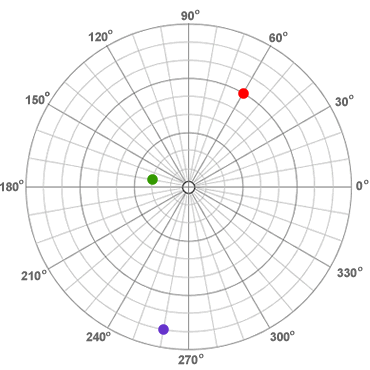Home » Free Printables » Printable Graph Paper » Polar Graph Paper

# Free Printable Polar Graph Paper

### What is Polar Graph Paper?

Polar graph paper, also known as polar coordinate paper, is a graph paper with concentric circles (with equal spacing between them) that are divided into small arcs. In plotting on this paper, one measures a radial distance from the pole (origin) and then rotates an angle of theta degrees from the polar axis (positive x axis).

In polar coordinates, the point (r, ?) is described as being a certain distance (r) from the pole (origin) and at a certain angle (?) from the polar axis (positive horizontal axis).

### Plotting Example

The polar coordinates graph below demonstrates the plotting of three points. The red point has polar coordinates of (6, 60o). The green point has polar coordinates of (2, 170o). And, the purple point has polar coordinates of (8, 260o).### About our Polar Coordinates Paper

We have four styles of polar coordinates graph paper...

-- six inch graph, black ink, one chart
-- four inch graph, black ink, two charts
-- six inch graph, blue ink, one chart
-- four inch graph, blue ink, two charts

Choose a size and an ink color that will work well with your plot. These charts print on a standard sheet of 8 1/2 x 11 paper. They can be opened and printed with a .pdf document reader such as Adobe Reader.

### More about Polar Coordinates Paper

 Free Printable Graph Paper, Our collection of twenty different graph paper designs that you can print and use for free. WaterproofPaper.com.

 Polar Coordinate System, Summary article about the polar coordinate system. Wikipedia article, last accessed 05/2020.

 Coordinate Converter, A calculator that allows you to convert between Cartesian, polar, and cylindrical coordinates. Random-Science-Tools.com interactive, last accessed 05/2020.

 Polar Coordinates, A short article with example plots and problems that demonstrate the polar coordinate system. Interactive Mathematics website article, last accessed 05/2020.